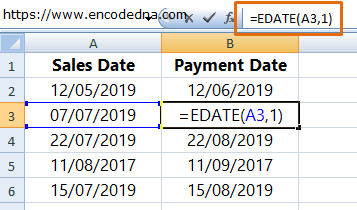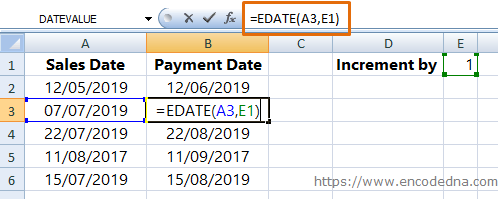﻿ Automatically add One Month from Previous Date in Excel

# Automatically add One Month from Previous Date in Excel

← Prev

In Excel, you can use the EDate() method to automatically add one month from the previous date or increment the previous date by one month.

Let us assume I have a date column (Sales Date), where I have a list of date in dd/mm/yyyy format. There’s another column (Payment Date), where I want to show date, which is one month plus from the previous date (or the Sales Date). See the image.The formula is clearly visible in the above image. To add the formula simply type,

=EDate(A3, 1), where A3 is cell 3 in the first column. Drag the formula down to other rows.

The method EDate() takes two parameters.

1. start_date: The date, which you want to increment by one month
2. months: The number of months you want to increment the date

You can hard code the value for month, like 1, 2, 3 etc. depending on the number of months you want to increment. Alternatively, the method can take value for the month from another column. For example,The EDate() method will show the incremented month irrespective of the date format you are using. Change the date format and see if it works.

## Thought you should know

You can assign a negative number value like -2 for the months parameter in the EDate() to get previous month or decrement the month from a given date. For example,

if a date is 27/07/2019, and you want to decrement it by 2 months, then formula will be,

=EDATE("12/05/2019", -2), the result is 12/06/2019

Or,

=EDate (A1, -2)